# How to Get the Maximum or Minimum Absolute Value in Excel

It is easy to find the maximum or minimum value in a batch of data in excel, but if this batch of numbers contains both positive and negative numbers, the maximum or minimum absolute value cannot be found out by basic MAX or MIN function in excel. This article will help you to solve this issue by using ABS function.

Prepare a table of numbers contains both positive and negative numbers: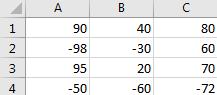As we all know, compare the absolute values for positive number and negative number, actually we compare the values without negative sign, so`|-11|` is larger than `|10|`. How can we find out the maximum or minimum value among the positive and negative numbers? Please follow below steps.

## Get the Maximum or Minimum Absolute Value in Excel

Step 1: Select a blank cell, enter the formula =MAX(ABS(A1:C4)).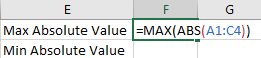Step 2: Press Ctrl + Shift + Enter together, then the MAX absolute value is displayed.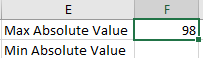Step 3: Use the similar formula to get the MIN absolute value. Enter the formula =MIN(ABS(A1:C4)).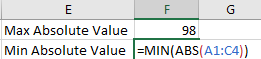Step 4: Press Ctrl + Shift + Enter together, then the MIN absolute value is displayed.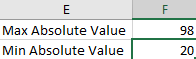## Get the Maximum or Minimum Absolute Value by User Defined Function

We can also define a function like MINABS or MINABS then we can directly use it to find out the MAX or MIN absolute value. See steps below.

Step 1: Click Developer tab->Visual Basic or Alt+F11 to load Microsoft Visual Basic for Applications window.

Step 2: Click Insert->Module to insert a module.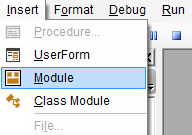Step 3: Enter below code in Module window.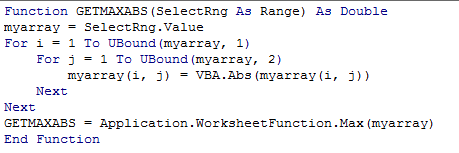```Function GETMAXABS(SelectRng As Range) As Double
myarray = SelectRng.Value
For i = 1 To UBound(myarray, 1)
For j = 1 To UBound(myarray, 2)
myarray(i, j) = VBA.Abs(myarray(i, j))
Next
Next
GETMAXABS = Application.WorksheetFunction.Max(myarray)
End Function```

Notes:

1. We define the function GETMAXABS to get the maximum absolute value in excel.
2. We can use the similar code to define another function GETMINABS to get the minimum absolute value as well. Just replace ‘max’ to ‘min’ in above code.

Step 4: Now we can try the user defined function GETMAXABS. After entering =get, we can find that defined function GETMAXABS is loaded properly.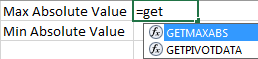Step 5: Enter =GETMAXABS(A1:C4).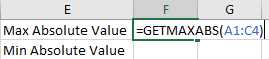Step 6: This time click Enter. Verify that MAX absolute value is displayed.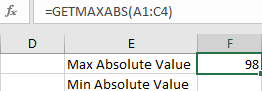### Related Functions

• Excel ABS Function
The Excel ABS function returns the absolute value of a number.The ABS function is a build-in function in Microsoft Excel and it is categorized as a Math and Trigonometry Function.The syntax of the ABS function is as below:=ABS (number)…
• Excel MIN function
The Excel MIN function returns the smallest numeric value from the numbers that you provided. Or returns the smallest value in the array.The MIN function is a build-in function in Microsoft Excel and it is categorized as a Statistical Function.The syntax of the MIN function is as below:= MIN(num1,[num2,…numn])….
• Excel MAX function
The Excel MAX function returns the largest numeric value from the numbers that you provided. Or returns the largest value in the array.= MAX(num1,[num2,…numn])…
Related Posts

How to Extract First Letter from Each Word in a Cell in Excel

This post will guide you how to extract first letter from each word in a given cell in Excel. How do I extract the first letter of each word in a range of names in your current worksheet in Microsoft ...

How to ignore Blank Cells while Concatenating cells in Excel

This post will guide you how to concatenate cells but ignore all blank cells in your worksheet in Excel. How do I concatenate cells but ignore blank cells with a formula in Excel. How to create a concatenate formula to ...

How to Calculate the Average Excluding the Smallest & Highest Numbers in Excel

Calculating the average for a batch of data is frequently used in our daily life. But for some cases like statistic the average score in a competition, or price analysis, we often calculate the average excluding the smallest and highest ...

How to Count Only Numbers in Bold in a Range of Cells in Excel

This post will guide you how to Count only numbers with bold style in a range of cells in Excel. How do I Count on cells with bold font within a range of cells using User defined function in Excel ...

How to Sum Only Numbers in Bold in a Range of Cells in Excel

This post will guide you how to sum only numbers with bold style in a range of cells in Excel. How do I sum on cells with bold font within a range of cells using User defined function in Excel ...

How to Filter Formula in Excel

This post will guide you how to filter your data with an Formula in Excel. How do I filter cells with formulas through a User defined function in Excel 2013/2016. Filter Formula Assuming that you have a list of data ...

How to Count Cells by Font Color in Excel

This post will guide you how to count cells by font color in Excel. How do I Count the number of cells with specific cell Font color by using a User Defined function in Excel. Count Cells by Font Color ...

How to Highlight Cells Containing Formulas in Excel

This post will guide you how to highlight cells in which contain formulas using Conditional Formatting feature in Excel. How do I conditionally format a cell if it contains formula using a User defined function in combination with Conditional Formatting ...

How to Extract Number from Text String in Excel

This post will guide you how to extract number from a given test string in Excel. How do I extract all numbers from string using a formula in Excel. How to get all number from a given test string using ...

How to Filter Data with Strikethrough Format in Excel

This post will guide you how to filter or delete rows with strikethrough format in your selected range of cells in Excel. How do I Filter by strikethrough formatted text with Find and Replace function in Excel 2013/2016. Or how ...

Sidebar